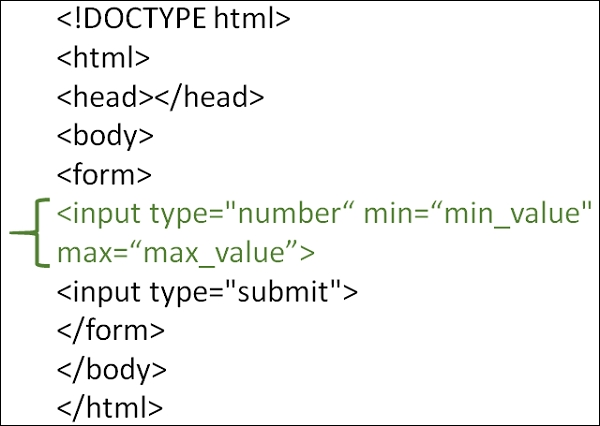# How to limit an HTML input box so that it only accepts numeric input?

HTMLWeb DevelopmentFront End Technology

To limit an HTML input box to accept numeric input, use the <input type="number">. With this, you will get a numeric input field.

After limiting the input box to number, if a user enters text and press submit button, then the following can be seen “Please enter a number.”## Example

You can try to run the following code to limit an HTML input box so that it only accepts numeric input −

<!DOCTYPE html>
<html>
</html>